# WO2013076937A1 - Ac conversion circuit - Google Patents

## Info

Publication number
WO2013076937A1
WO2013076937A1 PCT/JP2012/007333 JP2012007333W WO2013076937A1 WO 2013076937 A1 WO2013076937 A1 WO 2013076937A1 JP 2012007333 W JP2012007333 W JP 2012007333W WO 2013076937 A1 WO2013076937 A1 WO 2013076937A1
Authority
WO
WIPO (PCT)
Prior art keywords
switching
unit
voltage
phase
ac voltage
Prior art date
Application number
PCT/JP2012/007333
Other languages
French (fr)
Japanese (ja)
Inventor

Original Assignee
パナソニック株式会社
Priority date (The priority date is an assumption and is not a legal conclusion. Google has not performed a legal analysis and makes no representation as to the accuracy of the date listed.)
Filing date
Publication date
Priority to JP2011254791 priority Critical
Priority to JP2011-254791 priority
Application filed by パナソニック株式会社 filed Critical パナソニック株式会社
Publication of WO2013076937A1 publication Critical patent/WO2013076937A1/en

## Images

•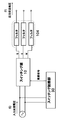•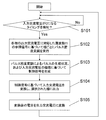•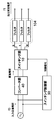•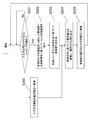•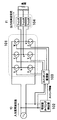•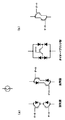••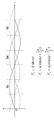•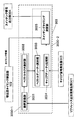•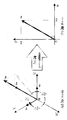•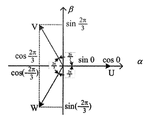••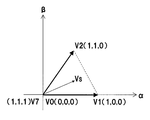•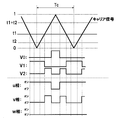•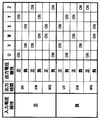•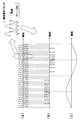•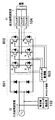•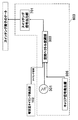•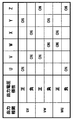•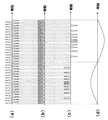•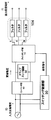•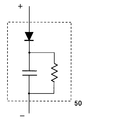•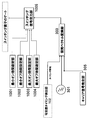•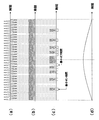•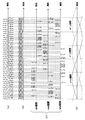•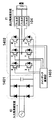••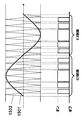## Classifications

• HELECTRICITY
• H02GENERATION; CONVERSION OR DISTRIBUTION OF ELECTRIC POWER
• H02MAPPARATUS FOR CONVERSION BETWEEN AC AND AC, BETWEEN AC AND DC, OR BETWEEN DC AND DC, AND FOR USE WITH MAINS OR SIMILAR POWER SUPPLY SYSTEMS; CONVERSION OF DC OR AC INPUT POWER INTO SURGE OUTPUT POWER; CONTROL OR REGULATION THEREOF
• H02M5/00Conversion of ac power input into ac power output, e.g. for change of voltage, for change of frequency, for change of number of phases
• H02M5/40Conversion of ac power input into ac power output, e.g. for change of voltage, for change of frequency, for change of number of phases with intermediate conversion into dc
• H02M5/42Conversion of ac power input into ac power output, e.g. for change of voltage, for change of frequency, for change of number of phases with intermediate conversion into dc by static converters
• H02M5/44Conversion of ac power input into ac power output, e.g. for change of voltage, for change of frequency, for change of number of phases with intermediate conversion into dc by static converters using discharge tubes or semiconductor devices to convert the intermediate dc into ac
• H02M5/453Conversion of ac power input into ac power output, e.g. for change of voltage, for change of frequency, for change of number of phases with intermediate conversion into dc by static converters using discharge tubes or semiconductor devices to convert the intermediate dc into ac using devices of a triode or transistor type requiring continuous application of a control signal
• H02M5/458Conversion of ac power input into ac power output, e.g. for change of voltage, for change of frequency, for change of number of phases with intermediate conversion into dc by static converters using discharge tubes or semiconductor devices to convert the intermediate dc into ac using devices of a triode or transistor type requiring continuous application of a control signal using semiconductor devices only
• HELECTRICITY
• H02GENERATION; CONVERSION OR DISTRIBUTION OF ELECTRIC POWER
• H02MAPPARATUS FOR CONVERSION BETWEEN AC AND AC, BETWEEN AC AND DC, OR BETWEEN DC AND DC, AND FOR USE WITH MAINS OR SIMILAR POWER SUPPLY SYSTEMS; CONVERSION OF DC OR AC INPUT POWER INTO SURGE OUTPUT POWER; CONTROL OR REGULATION THEREOF
• H02M5/00Conversion of ac power input into ac power output, e.g. for change of voltage, for change of frequency, for change of number of phases
• H02M5/02Conversion of ac power input into ac power output, e.g. for change of voltage, for change of frequency, for change of number of phases without intermediate conversion into dc
• H02M5/04Conversion of ac power input into ac power output, e.g. for change of voltage, for change of frequency, for change of number of phases without intermediate conversion into dc by static converters
• H02M5/22Conversion of ac power input into ac power output, e.g. for change of voltage, for change of frequency, for change of number of phases without intermediate conversion into dc by static converters using discharge tubes with control electrode or semiconductor devices with control electrode
• H02M5/275Conversion of ac power input into ac power output, e.g. for change of voltage, for change of frequency, for change of number of phases without intermediate conversion into dc by static converters using discharge tubes with control electrode or semiconductor devices with control electrode using devices of a triode or transistor type requiring continuous application of a control signal
• H02M5/297Conversion of ac power input into ac power output, e.g. for change of voltage, for change of frequency, for change of number of phases without intermediate conversion into dc by static converters using discharge tubes with control electrode or semiconductor devices with control electrode using devices of a triode or transistor type requiring continuous application of a control signal for conversion of frequency
• HELECTRICITY
• H02GENERATION; CONVERSION OR DISTRIBUTION OF ELECTRIC POWER
• H02MAPPARATUS FOR CONVERSION BETWEEN AC AND AC, BETWEEN AC AND DC, OR BETWEEN DC AND DC, AND FOR USE WITH MAINS OR SIMILAR POWER SUPPLY SYSTEMS; CONVERSION OF DC OR AC INPUT POWER INTO SURGE OUTPUT POWER; CONTROL OR REGULATION THEREOF
• H02M7/00Conversion of ac power input into dc power output; Conversion of dc power input into ac power output
• H02M7/42Conversion of dc power input into ac power output without possibility of reversal
• H02M7/44Conversion of dc power input into ac power output without possibility of reversal by static converters
• H02M7/48Conversion of dc power input into ac power output without possibility of reversal by static converters using discharge tubes with control electrode or semiconductor devices with control electrode
• H02M7/4826Conversion of dc power input into ac power output without possibility of reversal by static converters using discharge tubes with control electrode or semiconductor devices with control electrode operating from a resonant DC source, i.e. the DC input voltage varies periodically, e.g. resonant DC-link inverters

## Abstract

This AC conversion circuit converts a single-phase AC input voltage with a given frequency (f0) to a three-phase AC output voltage with a lower frequency (f1). This AC conversion circuit is provided with the following: a switching unit (101) that has a plurality of switching elements; and a switching control unit (103) that controls the conduction state of each switching element. The switching control unit (103) performs the conversion to a three-phase AC output voltage by inputting, to the switching unit (101), pulses that are pulse-density modulated on the basis of spatial vector modulation.

## Description

AC conversion circuit

Application, an AC voltage of a relatively high frequency, a technique for converting an AC voltage of relatively low frequency.

Recently, as a system for transferring power in a contactless power transfer system using a resonant magnetic field coupling is proposed. Patent Document 1 uses the electromagnetic coupling phenomenon between the two resonators, discloses a new wireless energy transmission device for transmitting energy through space. In the wireless energy transmission device, by coupling the two resonators through seepage of vibration energy of the resonant frequency occurring in the space around the resonator (evanescent tail), the vibration energy in a wireless (contactless) to transmission.

The output power of the resonator in the wireless power transmission system is an AC power having a frequency equal to the resonant frequency, the resonant frequency is usually set to at least 100kHz. When using the high frequency AC power as a general household power needs to be converted into AC power of low frequency 50 / 60Hz for use in the system power supply. In the case of performing rotation control of a direct motor or the like, it is necessary to convert the output frequency required.

On the other hand, as a technique for converting AC power of a constant frequency AC power of any frequency, there is inverter technology. Patent Document 2 discloses a general inverter technology. Its conversion method, once the AC power input is converted into DC power, and obtains AC power by changing thereafter cut the direction of the current to the load by using a plurality of switching elements. At this time, the output frequency is determined by the switching frequency of the switching element.

U.S. Patent Application Publication No. 2008/0278264 Pat JP 11-346478 discloses JP 4-79770 discloses US Pat. No. 6313602

In the conventional AC converter, because the high frequency AC power is temporarily converted into DC power, a loss of power occurs. Also, switch on in a state where the DC voltage is applied, because the off operation is performed, the switching loss. Furthermore, a capacitor for rectifying is required, problems of reduction in cost and an increase in durability occurs.

The present disclosure, the AC power of a relatively high frequency input from the wireless power transmission system or the like, to provide an AC conversion circuit capable of suppressing a decrease in conversion efficiency in converting into AC power of a relatively low frequency.

In order to solve the above problems, the AC conversion circuit according to an embodiment of the present disclosure, the input AC voltage of a single phase of the frequency f0, converts the output AC voltage of three phases of the frequency f1 is lower than the frequency f0 AC a conversion circuit to convert the input AC voltage based on a control signal, the converted voltage, and a switching unit for outputting the phase that is selected on the basis of the control signal, the high frequency component of the voltage of the converted by removing a filter unit for converting the voltage of the converted into the output AC voltage, in synchronization with the timing of the input AC voltage becomes zero, the reference frequency f1 corresponding to each phase of the output AC voltage It performs pulse density modulation in each phase based on the signal to generate the control signal based on the polarity of the pulse density modulation by pulse generation status, and the input AC voltage, the control signal The and a switching control unit for sending to the switching unit. The switching unit, the polarity of the input AC voltage and the first type of switching elements for applying a positive voltage for positive to the corresponding phase, the polarity of the input AC voltage corresponds to a positive voltage when the negative and a second type of switching elements to be applied to the phase. The switching control unit, polarity positive of the input AC voltage, and when the polarity of the reference signal in the pulse density modulation is positive, turn on the first type of switching elements, of the input AC voltage If the negative polarity is, and the polarity of the reference signal in the pulse density modulation is positive, turn on the second type of switching elements, the polarity of the input AC voltage is positive, and the in the pulse density modulation when the polarity of the reference signal is negative, and turning on the second type of switching elements, a negative polarity of said input AC voltage, and when the polarity of the reference signal in the pulse density modulation is negative, Turn on the first type of switching elements, one of said first type of switching elements and the second switching element, but corresponding to the same phase So as not to turn towards the same time, it outputs the control signal for controlling the switching unit. The switching control unit performs the pulse density modulation based on the spatial vector modulation.

Other AC converter according to an embodiment of the present disclosure, an AC conversion circuit an input AC voltage of a single phase of the frequency f0, converts the three-phase AC output voltage of the frequency f0 lower frequency f1 than the a converter unit for converting an input AC voltage into a DC voltage, a switching unit converts the DC voltage based on the control signal, and outputs the converted voltage, the phase that is selected on the basis of the control signal, the conversion by removing high-frequency components of the voltage after a filter unit for converting the voltage of the converted into the output AC voltage, in synchronization with the timing of the input AC voltage becomes zero, the phase of the output AC voltage It performs pulse density modulation in each phase on the basis of a reference signal of a frequency f1 corresponding to generate the control signal based on the pulse generation conditions of by the pulse density modulation, the switching unit And a switching control unit for sending. The switching unit, the polarity of the input AC voltage and the first type of switching elements for applying a positive voltage for positive to the corresponding phase, the polarity of the input AC voltage corresponds to a positive voltage when the negative and a second type of switching elements to be applied to the phase. The switching control unit, when the polarity of the reference signal in the pulse density modulation is positive, the first type of switching elements is turned on, when the polarity of the reference signal in the pulse density modulation is negative , the second type of switching elements is turned on, one of the first type of switching elements and the second switching element, so as not to turn on both of those corresponding to the same phase at the same time, the switching and it outputs the control signal for controlling the parts. The switching control unit performs the pulse density modulation based on the spatial vector modulation.

General and specific aspects of the above-described systems, methods, and are implemented using a computer program, or system may be implemented using a combination of methods and computer programs.

According to an embodiment of the present disclosure, since the input voltage of the high-frequency AC power input switching operation is performed at zero, it is possible to power conversion with high efficiency than conventional.

Is a diagram illustrating an example of a schematic configuration of an AC conversion circuit in the exemplary embodiment. Is a flow chart showing an example of the operation of the AC conversion circuit in the exemplary embodiment. It is a diagram showing another example of a schematic configuration of an AC conversion circuit in the exemplary embodiment. It is a flow chart showing another example of the operation of the AC conversion circuit in the exemplary embodiment. It is a diagram showing a configuration of an AC conversion circuit according to the first embodiment. It is a diagram illustrating a configuration example of a switching element in the first embodiment. Is a diagram showing the configuration of a switching control unit in the first embodiment. Is a diagram illustrating an example of a three-phase sine wave output from the reference sinusoidal wave generating unit. It is a diagram showing a configuration of a space vector modulation section in the first embodiment. It is a diagram for explaining the principle of the space vector modulation. It is a diagram showing the relationship between the three-phase coordinate system and the two-phase coordinate system. It is a diagram showing eight switching vectors and six sectors. Is a diagram illustrating an example of a command vector Vs in sector 1. Is a diagram illustrating an example of a method for determining the switching state by comparison between the carrier signal and the duration of each switching vector. It is a diagram showing the relationship between the switch to output polarity and on the first embodiment. It is a diagram showing a waveform of each voltage in the first embodiment. (A) is a diagram showing the waveform of the input AC voltage, (b) is a diagram showing an output waveform of the switching unit is a diagram showing a (c) an output waveform of the filter. It is a diagram showing a configuration of an AC conversion circuit according to the second embodiment. Is a diagram showing the configuration of a switching control unit in the second embodiment. Is a diagram showing the relationship between the switch to output polarity and on in the second embodiment. It is a diagram showing a waveform of each voltage in the second embodiment. (A) is a diagram showing the waveform of the input AC voltage, (b) is a diagram showing an output waveform of the converter circuit, (c) is a diagram showing an output waveform of the switching unit, (d) the filter is a diagram showing an output waveform. Is a configuration diagram in which a clamp snubber circuit. It is a diagram illustrating a configuration example of a clamp snubber circuit. Is a diagram showing the configuration of a switching control unit in the third embodiment. It is a diagram showing a waveform of each voltage in the third embodiment. (A) is a diagram showing the waveform of the input AC voltage, (b) is a diagram showing an output waveform of the converter circuit, (c) is a diagram showing an output waveform of the switching unit, (d) the filter is a diagram showing an output waveform. Always it illustrates input and output waveforms in the first embodiment to output a power in only one phase. (A) is a diagram showing the waveform of the input AC voltage, (b) is a diagram showing an output waveform of the converter circuit, (c) is a diagram showing an output waveform of the switching unit, (d) each is a diagram showing an output waveform of the filter phase. It is a diagram showing a configuration of a conventional AC converter. Is a diagram showing the configuration of a switching controller of the conventional AC converter. It is a diagram illustrating a switching timing of the switching control unit of the conventional AC converter.

Outlining one exemplary embodiment of the present disclosure is as follows.

AC conversion circuit according to an embodiment of the present disclosure, an AC conversion circuit an input AC voltage of a single phase of the frequency f0, converts the three-phase AC output voltage of the frequency f0 lower frequency f1 than the control signal converting said input AC voltage based on the converted voltage, and a switching unit for outputting the phase that is selected on the basis of the control signal, by removing the high frequency component of the voltage of the converted, the conversion a filter unit for converting the voltage after the output AC voltage, in synchronization with the timing of the input AC voltage becomes zero, pulse every phase on the basis of a reference signal of a frequency f1 corresponding to each phase of the output AC voltage It performs density modulation, and generates the control signal based on the polarity of the pulse density modulation by pulse generation status, and the input AC voltage, and sends the control signal to the switching unit And a switching control unit. The switching unit, the polarity of the input AC voltage and the first type of switching elements for applying a positive voltage for positive to the corresponding phase, the polarity of the input AC voltage corresponds to a positive voltage when the negative and a second type of switching elements to be applied to the phase. The switching control unit, polarity positive of the input AC voltage, and when the polarity of the reference signal in the pulse density modulation is positive, turn on the first type of switching elements, of the input AC voltage If the negative polarity is, and the polarity of the reference signal in the pulse density modulation is positive, turn on the second type of switching elements, the polarity of the input AC voltage is positive, and the in the pulse density modulation when the polarity of the reference signal is negative, and turning on the second type of switching elements, a negative polarity of said input AC voltage, and when the polarity of the reference signal in the pulse density modulation is negative, Turn on the first type of switching elements, one of said first type of switching elements and the second switching element, but corresponding to the same phase So as not to turn towards the same time, it outputs the control signal for controlling the switching unit. The switching control unit performs the pulse density modulation based on the spatial vector modulation.

AC converter according to another aspect of the present disclosure is an AC converter circuit that an input AC voltage of a single phase of the frequency f0, converts the three-phase AC output voltage of the frequency f0 lower frequency f1 than the a converter unit for converting an input AC voltage into a DC voltage, a switching unit converts the DC voltage based on the control signal, and outputs the converted voltage, the phase that is selected on the basis of the control signal, the conversion by removing high-frequency components of the voltage after a filter unit for converting the voltage of the converted into the output AC voltage, in synchronization with the timing of the input AC voltage becomes zero, the phase of the output AC voltage It performs pulse density modulation in each phase on the basis of a reference signal of a frequency f1 corresponding to generate the control signal based on the pulse generation conditions of by the pulse density modulation, sent to the switching unit And a switching control unit for. The switching unit, the polarity of the input AC voltage and the first type of switching elements for applying a positive voltage for positive to the corresponding phase, the polarity of the input AC voltage corresponds to a positive voltage when the negative and a second type of switching elements to be applied to the phase. The switching control unit, when the polarity of the reference signal in the pulse density modulation is positive, the first type of switching elements is turned on, when the polarity of the reference signal in the pulse density modulation is negative , the second type of switching elements is turned on, one of the first type of switching elements and the second switching element, so as not to turn on both of those corresponding to the same phase at the same time, the switching and it outputs the control signal for controlling the parts. The switching control unit performs the pulse density modulation based on the spatial vector modulation.

In certain embodiments, the alternating current conversion circuit has a snubber circuit for reducing the unwanted oscillation at the time of switching between the converter unit and the switching unit.

In certain embodiments, the snubber circuit includes a diode and a capacitor resistor and.

In certain embodiments, the switching control unit, in synchronism with the timing of the input AC voltage becomes zero, the reference signal generating unit to generate three types of reference signals in the frequency f1 corresponding to each phase of the output AC voltage a carrier signal generating unit for generating a carrier signal of a frequency higher than the frequency of the reference signal, the three types of reference signals generated by said reference signal generating unit, and the carrier generated by the carrier signal generating unit based on the signal, and a space vector modulation section for performing spatial vector modulation. The space vector modulation section, on the basis of the three types of reference signals, to determine the conductive state of each switching element in the switching unit, by using the carrier signal, determines the duration of the conducting state of the switching elements .

In certain embodiments, the space vector modulation section, the three types of reference signals is converted into a pulse by the spatial vector modulation, switching element corresponding to the pulse of each phase at a timing at which the value of the input AC voltage becomes 0 and outputs it to.

In certain embodiments, the switching control unit, based on the input and determining positive or negative judgment unit the polarity of the AC voltage, wherein the pulse outputted from the space vector modulation section, and the sign determination of the determination result, the control It generates a signal, and a switching signal output unit for sending to the switching unit.

In certain embodiments, the switching control unit switches the ON and OFF states of each switching element at a predetermined minimum on-time and minimum off-hours or time interval was, the minimum on-time and the minimum off time, n1 / 2f0 (n1 is an integer of 2 or more) are set to.

In certain embodiments, the switching control unit switches the ON and OFF states of each switching element at a preset maximum on-time and maximum OFF time or less time interval was, the maximum on-time and the maximum off time, n2 / 2f0 (n2 is an integer of 2 or more) are set to.

In certain embodiments, the switching control unit, the voltage of the half cycle of the input AC voltage is always controls the switching unit so as to be outputted to only one particular phase.

In certain embodiments, the AC conversion circuit detects the timing at which the value of the input AC voltage becomes zero, further comprising a zero cross timing detection unit notifies the switching control unit.

Before describing the specific embodiments of the present disclosure, firstly, an outline of the technology in the present inventors have found a problem and the present disclosure the conventional art.

Figure 20 is a wireless power transmission system frequency single-phase alternating current, such as using conventional inverter technology is a configuration diagram of a power receiving side AC converter that converts more three-phase alternating current of low frequency. The AC conversion circuit includes a rectification unit 1401 which converts the input high-frequency AC power into DC power, an inverter unit 1402 for outputting the output voltage of the rectifier unit 1401 in each phase of a plurality of switching elements, it is arranged for each phase low-pass filter unit 104 (hereinafter, "filter") and a. AC converter further includes a switching control unit 1403 for controlling the operation of the plurality of switching elements included in the inverter unit 1402.

Hereinafter, the operation of the AC conversion circuit shown in FIG. 20. First, high-frequency AC power input is converted into DC power in the rectifier section 1401. Next, the inverter unit 1402, the switching elements U as the direction of the current flowing to each phase of the load is switched alternately, V, W, X, Y, Z on and off is switched. Here, the switching elements U, V, W, X, Y, Z, generally to semiconductor switches MOSFET or IGBT or the like is used. On of the switching elements, the timing control of the off pulse width modulation (PWM: Pulse Width Modulation) is used.

Figure 21A, 21B is a diagram for explaining the configuration and operation of the switching control unit 1403. As shown in FIG. 21A, the switching control unit 1403, the input of low-frequency power of the reference sine wave 1501 is set to the same frequency as the triangular wave 1502 and preset to a frequency higher than the frequency to be output and a PWM control section 1503 which receives the. PWM control unit 1503, a pulse generated based on the reference sine wave 1501 and a triangular wave 1502, is input to the gate of a predetermined switching element.

Here, as an example, an operation of the switching control unit 1403 in the case of outputting the power between uv phase. 21B is a diagram illustrating an example of switching timing by the PWM control section 1503. First, PWM control unit 1503 compares the respective input values ​​of the reference sine wave 1501 and a triangular wave 1502. In the case of "reference sinusoidal ≧ 0" and "reference sinusoidal ≧ triangular wave", check the switching elements U and the switching element Y, "reference sinusoidal ≧ 0" and "reference sinusoidal <triangular wave" in the case of turns off the switching elements U and the switching element Y. In the case of "reference sinusoidal <0" and "reference sinusoidal ≧ triangular wave" turns off the switching elements V and the switching element X, "reference sinusoidal <0" and "reference sine wave < in the case of triangular wave "turns on the switching elements V and the switching device X. By this operation, the width of pulses output from the PWM controller 1503 changes according to the magnitude of the reference sine wave value.

DC power input to the inverter unit 1402, by the above switching operation, is converted into a pulse train having the same width and the pulse shown in FIG. 21B, are output. The output pulse train, by passing through the low-pass filter unit 104, as the final output is converted to a sine wave of a desired frequency. Here, although has been described as an example a configuration to obtain a sinusoidal output, by the reference sine wave to an arbitrary frequency and waveform, the AC power having a high frequency AC power input any frequency and waveform It can be converted to.

However, the AC conversion circuit constructed as described above, in the rectifier unit 1401, since the high-frequency AC power is temporarily converted into DC power, a loss of power occurs. Also in inverter section 1402, switch on in a state where the DC voltage is applied, because the off operation is performed, the switching loss. Furthermore, a capacitor for rectifying is required, problems of reduction in cost and an increase in durability occurs.

The present inventors have newly found the above-mentioned problems, reduction in the conversion efficiency in converting the AC power of a relatively high frequency input from the wireless power transmission system or the like, into AC power of a relatively low frequency the completed the AC conversion circuit can be suppressed. Hereinafter, an outline of embodiments of the present disclosure.

Figure 1A is a diagram illustrating a configuration example of an AC conversion circuit according to one embodiment of the present disclosure. AC converting circuit shown is a single-phase AC voltage of a frequency f0 (hereinafter sometimes referred to as "input AC voltage.") And three-phase AC voltage of lower frequency f1 than the frequency f0 (hereinafter, " it is configured to convert sometimes referred to as an output AC voltage ".). The AC conversion circuit converts an input AC voltage based on a control signal, the voltage of each phase after conversion (uv interphase, vw phases, wu interphase) and switching unit 10 for outputting the high frequency from the output of the switching unit 10 and a filter unit 104 for outputting an output AC voltage by removing the component. AC converter also comprises a switching control unit 30 for controlling the switching unit 10 by generating the control signal, and inputs to the switching unit 10.

The switching control unit 30, in synchronization with the timing at which the input AC voltage becomes zero, performs pulse density modulation in each phase on the basis of a reference signal of a frequency f1 corresponding to each phase of the output AC voltage. Then, a control signal is generated based on the polarity of the pulse generation status, and the input AC voltage by pulse density modulation, and sends to the switching unit 10. Whether to output the converted voltage to which phase This control signal is selected. The above operation, each time the input AC voltage becomes zero, that is performed for each half cycle of the input AC voltage. Here, "pulse generation status of" means for example a pulse generator whether or of each phase, the polarity or the like of the generated pulse.

The switching unit 10 typically has a plurality of switching elements, on the particular switching device that is selected by the control signal, by switching off and distributes an input voltage into three-phase. Here, the "ON" represents a conductive state, the "OFF" represent the non-conducting state. With this configuration, dynamically it is possible to generate the desired three-phase alternating current depending on the polarity of the polarity and the output AC voltage of the input AC voltage. Note that "input AC voltage is zero" is not limited to the case of completely becomes 0, is intended to include the range that can be regarded as substantially zero. In the present specification, the value for the amplitude of the input AC voltage, if contained in the range of less than 10%, it is assumed substantially zero.

Figure 1B is a flow chart showing a flow of operation of the AC conversion circuit shown in FIG. 1A. First, in step S101, the timing at which the input AC voltage becomes zero is detected. Step S101 is executed, for example, by a detector (not shown). If the timing of the input AC voltage becomes zero is detected, the process proceeds to step S102, pulse density modulation is performed for each phase on the basis of a reference signal of a frequency f1 corresponding to each phase of the output AC voltage. Subsequently, in step S103, the control signal is generated based on the polarity of the pulse generation status, and the input AC voltage by pulse density modulation. Step S102 and S103 are executed by the switching control section 30. Thereafter, in step S104, the switching unit 10 based on the control signal, converts the input AC voltage, and outputs the phase to the selected converted voltage. Finally, in step S105, the filter unit 104 converts the converted voltage into output AC voltage. By the above operation is repeated for each half cycle of the input AC voltage, input AC voltage is converted to a relatively low frequency of the output AC voltage.

In this example, the control signal is to be sent to the switching unit 10 in synchronization with the timing at which the input AC voltage becomes zero, the switching operation performed by the internal switching unit 10, the voltage is performed in the state of zero. Therefore, it is possible to reduce the power loss due to the switching operation. Furthermore, in order to convert the output AC voltage without converting an input AC voltage into a DC voltage, it is possible efficient conversion. The switching unit 10, the detailed configuration and operation of such a switching control unit 30 will be described in the embodiment 1 described later.

AC converter is not limited to the above configuration, and may have other configurations. Figure 1C is a diagram showing another configuration example of an AC conversion circuit according to the present disclosure. The AC converter also an input AC voltage of a single phase of the frequency f0, is configured to convert three-phase AC output voltage of lower frequency f1 than the frequency f0. The AC conversion circuit, in addition to the components shown in FIG. 1A, and a converter 40 for input to the switching unit 10 further converts the input AC voltage once into a DC voltage.

Switching control unit 30 in this example also, in synchronization with the timing of the input AC voltage becomes zero, performs pulse density modulation in each phase on the basis of a reference signal of a frequency f1 corresponding to each phase of the output AC voltage. Then, a control signal is generated based on the pulse generation conditions of by the pulse density modulation, and sends to the switching unit. In this example, the converter section 40 converts the input AC voltage once into a DC voltage, the voltage input to the switching unit 10 is always positive. Therefore, the switching control unit 30 controls the switching unit 10 based on only the generation status of the pulse.

Figure 1D is a flow chart showing a flow of operations of the AC conversion circuit shown in FIG. 1C. In this configuration, the input AC voltage is converted into a DC voltage by the converter unit 40 in step S200. On the other hand, in step S201, the timing at which the input AC voltage becomes zero is detected. If the timing of the input AC voltage becomes zero is detected, the process proceeds to step S202, pulse density modulation is performed for each phase on the basis of a reference signal of a frequency f1 corresponding to each phase of the output AC voltage. Subsequently, in step S203, the control signal is generated based on the pulse generation conditions of by the pulse density modulation. After step S200 and S203 are completed, in step S204, the switching unit 10 based on the control signal, it converts the DC voltage output from the converter unit 40, and outputs the phase to the selected converted voltage. Finally, in step S205, the filter unit 104 converts the converted voltage into output AC voltage. Note that the step S200, the steps S201 S203, may be performed in parallel. By the above operation is repeated for each half cycle of the input AC voltage, input AC voltage is converted to a relatively low frequency of the output AC voltage.

In the structure shown in FIG. 1C, since the control signal in synchronization with a timing at which the input AC voltage becomes zero is sent to the switching unit 10, a switching operation performed by the internal switching unit 10 is performed in the state of the voltage 0 It is. Therefore, it is possible to reduce the power loss due to the switching operation. Note that the switching unit 10 in this example, the detailed configuration and operation of such a switching control unit 30 will be described in the second embodiment described later.

In the above description, each component of the AC converter is represented as a separate functional unit is blocked, the AC conversion circuit by executing a program that defines the processing of these functional units in the processor operations may be realized. Procedure of such a program, for example FIG. 1B, is shown in 1D.

Hereinafter will be described a more specific embodiment of the present disclosure. In the following description, it is denoted by the same reference numerals to the same or corresponding elements.

(Embodiment 1)
First, the AC conversion circuit according to the first embodiment. Figure 1E is a block diagram showing the schematic configuration of an AC conversion circuit of this embodiment. AC conversion circuit of this embodiment, an input AC voltage of a single phase of the frequency f0, is configured to convert three-phase AC output voltage of relatively low frequency f1. AC conversion circuit, the zero crossing timing detecting section 102 that detects a switching unit 101, the timing at which the value of the input AC voltage becomes 0 (zero cross timing) to output an input AC voltage by a plurality of switching elements in each phase, a switching control unit 103 for controlling the operation of each switching element, and a filter 104 to eliminate high frequency components of the output voltage of the switching unit 101. The subsequent filter 104 load is connected, an AC voltage with a frequency f1 is supplied to the load. Frequency f0 is set to, for example, more than 100kHz, the frequency f1 may be set to, for example, the same 50Hz frequency of the power system. Input AC voltage and the output AC voltage is assumed to be both sinusoidal voltage. Input AC voltage may be, for example, radio frequency (RF) voltage outputted from the power receiving unit of the wireless power transmission system utilizing the resonance magnetic field coupling.

The switching unit 101, a frequency f0 and single-phase AC voltage is input. The switching unit 101 includes switching elements U, which operates based on a control signal inputted from the switching control unit 103, V, W, X, Y, and Z. The switching elements U, V, W, X, Y, the Z, or the switching unit 101, uv phase, vw phase, to the subsequent filter 104 connected to each phase of the wu-phase, and outputs an input AC voltage switch the whether. Hereinafter, uv phase, vw phase, the wu-phase, for simplicity, u-phase, respectively, v-phase, may be referred to as a w-phase.

Switching elements U, V, W, the polarity of the input high-frequency AC is used to apply a positive voltage to the phase corresponding to the time of the positive, it may be referred to as a "first type of switch" herein. Switching elements X, Y, Z is a switch polarity of the input high-frequency AC is a positive voltage is applied to the phase corresponding to the time of the negative may be referred to as "second type of switch" herein.

Figure 2 is a diagram illustrating a configuration example of the switching elements. Each of the switching elements, for example, as shown in FIG. 2 (a), MOSFET or IGBT is a conventional semiconductor switching device, constructed and connected diodes in series or in parallel, the connected configuration to the diode in the bridge a. Alternatively, as shown in FIG. 2 (b), it may be constituted by a bidirectional switching element such as reverse blocking IGBT. The gates of the switching elements, the control signal is input from the switching control unit 103.

Next, specifically described the structure and operation of the switching control unit 103. Figure 3A is a diagram showing a specific configuration of the switching control unit 103. The switching control unit 103, each phase of the output three-phase sine wave of the AC voltage of the same frequency f1 as the reference sine wave generating unit 301 for generating (reference signal) (reference signal generating section), the input AC voltage polarity (positive or negative ) positive and negative determining section 302 determines a space vector modulation section 303 for generating a pulse train corresponding to each phase by a spatial vector modulation, the switching signal output unit 304 for outputting a control signal to be input to the gates of the switching elements, and a carrier signal generating section 305 that generates a signal as a reference for determining the switching times (carrier signal) in space vector modulation.

In the present embodiment, the pulse density modulation is performed using a modulation scheme called space vector modulation. Space vector modulation, for example, disclosed in Patent Documents 3 and 4. The entire disclosure of Patent Documents 3 and 4 incorporated herein by reference.

The space vector modulation section 303, a zero cross timing detector 102, timing information indicating a timing at which the voltage value of the input AC voltage becomes zero is input from the carrier signal generating section 305, the carrier signal. Also, the positive and negative determining section 302 is arranged to receive input from the input high-frequency AC line. Here, the carrier signal outputted from the carrier signal generating section 305 is, for example, a triangular wave of frequency fc. Frequency fc of the carrier signal is higher than the frequency f1 of the reference signal outputted from the reference sine wave generation section 301, and may be set lower than the frequency f0 of the input RF power. Frequency fc may be set so as to satisfy the F1«fc«f0.

Reference sine wave generation section 301 generates a three-phase sine wave of a sufficiently small electric power compared to the input high-frequency AC power, a frequency phase is shifted by 120 degrees (2π / 3) f1 (= 50Hz), input to the space vector modulation section 303 corresponding to each phase. Here, the output of the reference sine wave generator 301 is assumed to be a sine wave positive and negative values ​​around a zero.

Figure 3B is a diagram illustrating an example of a three-phase sine wave output from the reference sine wave generation section 301. Three-phase sinusoidal voltage Vu, Vw, Vv, respectively u phase, v phase, because it corresponds to the frequency and phase of the voltage to be output to the w-phase, their amplitudes are equal to each other, the phase is 2 [pi / 3 It is shifted by. Sine wave Vu, Vv, Vw can be respectively represented by Equations 1 to 3 below.

Here, the amplitude A1, the angular frequency is ω1 (= 2πf1), a sinusoidal voltage Vu of phase corresponding to the u-phase is the origin of the time the time coordinate becomes 0. The origin of the time coordinate may be determined at any time.

Space vector modulation section 303, the voltage value of the three-phase sine wave input Vu, Vv, as a command value Vw, performs spatial vector modulation. Then, a carrier signal inputted from the carrier signal generating section 305, based on the timing information input from the zero crossing timing detecting section 102, a pulse in synchronization with the timing at which the voltage level of the input AC voltage becomes zero switching signal Output and outputs it to the section 304.

Figure 3C is a diagram showing a configuration of a space vector modulation section 303. Space vector modulation section 303 includes a switching vector determination unit 3030-1 for determining a set of three vectors defining the combination of switching elements to be turned on based on the input from the reference sine wave generation section 301 (switching vector), set of time allocation of the determined vector and a switching state determining unit 3030-2 for determining the timing of the conduction state represented by (duty ratio) and each vector. Switching vector determination unit 3030-1 includes a coordinate conversion unit 3031 for converting the three-dimensional vector having the values ​​of the three-phase AC voltage input from the reference sine wave generator 301 and the components in a two-dimensional vector, a two-dimensional converted and a vector sector selection unit 3032 to determine the three switching vectors by selecting sectors vector belongs. Switching state determining unit 3030-2 includes a vector duty calculating section 3033 that calculates the duty of the three switching vector, a carrier duty comparator 3034 for comparing the duty and carrier signal, switching by the switching vectors based on the comparison result and a switching time determination unit 3035 for determining a time.

Hereinafter will be described a pulse density modulation process based on space vector modulation by the switching control unit 303.

First, a three-phase two-phase stationary coordinate transformation. Figure 4 is a conceptual view of a three-phase two-phase stationary coordinate transformation. Input value from the reference sine wave generation section 301 (three-phase balanced voltage instantaneous value) Vu, Vv, and Vw, as shown in FIG. 4 (a), respectively in the plane 2 [pi / 3 three different axes U, V, think W on the instantaneous vector Vu, Vv, to be Vw. Then, three-phase AC voltage input from the reference sine wave generator 301, i.e. the voltage to be output to each phase can be represented by combining instantaneous vector Vs of the formula 4 below.

This synthetic instantaneous vector Vs is a vector of constant magnitude that rotates at a constant angular frequency f1.

Then, the combined instantaneous vector, as shown in FIG. 4 (b), consider the α axis that matches the U-axis, that is expressed by β axis perpendicular thereto. The α component of Vs at this time V.alpha, the β component and V?.

Next, determine the conversion equation of three-phase two-phase stationary coordinate transformation. Figure 5 is a diagram showing the relationship between the three-phase coordinate system and the two-phase coordinate system. 5, U-axis, when the V axis, the vector on the W-axis, and the projection α axis, the β-axis shows Become any size. From FIG. 5, V.alpha, V? Can be expressed by Equation 5 below.

This is rewritten using the matrix, equation 6 is obtained. Here, for the relative conversion without changing the size of the converted voltage is multiplied by a constant 2/3 in the transformation matrix.

Coordinate conversion unit 3031, the input value from the reference sine wave generation section 301 (Vu, Vv, Vw) as a command value, based on the equation 6 above, the phase command value converting a three-phase two-phase stationary coordinate. This transformation, the voltage value of the three-phase sine wave input from the reference sine wave generator 301, can be treated as a constant rotating vector magnitude.

Then, by using this rotation vector, a method of determining the phases of the conducting state. Switching unit 101 shown in FIG. 1E, six switching elements U, V, W, X, Y, has the Z, of which, V corresponding to U and X, v-phase corresponding to the u-phase and Y , each pair of W and Z corresponding to the w-phase, one when on is controlled such other is turned off. Because of this limitation, combination of conduction states of all the switching elements, three switching elements U depicted in the upper side in FIG. 1E, V, is determined by a combination of W on and off. The combination is present are 2 3 = 8.

We define a vector space represented (U, V, W) in the state of each phase of the on-off. The case of the on-1, in the case of off to zero. For example, in the case where the U phase is ON (state in which power is supplied), V-phase, W-phase is off (no power is supplied), (U, V, W) = (1,0,0 ) and is described.

Figure 6 is similar to FIG. 4, the angle is three different 120 degrees from each other on a plane axes U, V, on coordinates represented by W, or plotted eight vector indicating the selectable switching states is there. These eight vectors, as shown in FIG. 6, V0 (0,0,0), V1 (1,0,0), V2 (1,1,0), V3 (0,1,0) , V4 (0,1,1), V5 (0,0,1), V6 (1,0,1), is represented as V7 (1,1,1). Here, V0 and V7, zero (zero) is a vector, V1, V3, V5 is a vector on each U, V, W axis. In the present specification, the vector V0 ~ V7, referred to as a "switching vector".

The region surrounded by the vector V0 ~ V7, angles think be divided into six sectors different by 60 degrees. As shown in FIG. 6 and Table 1, the vector V1 (1,0,0), V2 (1,1,0), the sector surrounded by V0 (0,0,0) is defined as sectors 1, wherein it is assumed that the shake the sector number in a counter-clockwise from.

Vector sector selection unit 3032 selects whether instruction value vector Vs (Vα, Vβ) as shown in FIG. 4 that has been converted by the coordinate conversion unit 3031 belongs to which sector to form a peripheral region of the sector selected 3 One vector as a vector indicating the switching state should be set, and outputs the result in the vector duty calculating section 3033. Note that the command value vector Vs (Vα, Vβ) Whether belongs to which sector, as shown in Table 1, is determined based on the phase θ of the vector Vs. The phase θ of the vector Vs, is determined by calculating the arctangent of the V? / V.alpha (arctangent).

Vector duty calculating section 3033 based on the selection result input from the vector sector selection unit 3032, calculates an output time ratio of the switching state represented by each vector (duty ratio). Hereinafter, a method for determining the output time ratio, the input sector describes a case of 1 as an example.

Figure 7 is a command value vector Vs when the sector number is 1, a diagram illustrating a vector that forms the peripheral region. If the sector number is 1, the vector should be selected, V1 (1,0,0), V2 (1,1,0), the V0 (0,0,0). Although in FIG. 7 is shown a vector V7 (1, 1, 1) it is also the vector because it means that outputs power to all phases, is not adopted in this embodiment. At this time, the instruction value vector Vs (Vα, Vβ), and considering that expressed by linear combination of the vectors V0, V1, V2. V0 = (V0α, V0β) = (0,0), V1 = (V1α, V1β) = (1,0,0), V2 = (V2α, V2β) = (1,1,0) and then, the carrier signal generator output time ratio of the vector V1 when one cycle of the carrier signal outputted from section 305 was 1 (duty) t1, and the output time ratio of the vector V2 t2. Then, the output time ratio t0 of vector V0 is a 1- (t1 + t2). That, t0 can be calculated by obtaining the t1 and t2.

At this time, the relationship between the elements of the output time ratio command value vector Vs of each vector can be expressed by Equation 7 below.

From Equation 7, when extracting only the expression for t1, t2, of formula 8 is obtained.

Here, the matrix A, defined by the following equation 9.

Then, t1, t2, from Cramer's rule, is represented by the formula 10 below.

Here, the determinant | A | is expressed by equation 11 below.

The operation of the above, it is possible to determine the t1, t2. Further, from t0 = 1-t1-t2, it can also be determined t0. Thus, the ratio of the duration of the switching state represented by three vectors V0, V1, V2 is determined. The above example has been assumed that the sector 1, the case of sectors 2-6, by the same processing, determination and the ratio of the duration of their switching state of the vector defining the switching state is determined.

Then, information indicating the duration ratio of a switching state corresponding to each output vector calculated by the vector duty calculating section 3033 is input to the carrier duty comparing section 3034. Carrier duty comparing section 3034, in terms of normalized such that the carrier signal inputted from the carrier signal generator 305 to a value between 0 and 1 per cycle, and duration ratios of the respective switching state input comparing the magnitude, and outputs the result to the switching time determining section 3035.

On the other hand, the zero-crossing timing detecting section 102 detects the timing at which the voltage value of the input AC voltage becomes zero, and notifies the space vector modulation section 303 the detected information as the timing information. Switching time determining section 3035 based on the comparison result and the timing information input, to determine the duration of each switching state.

Hereinafter, an example in which the sector 1 is selected, explaining the process of determining the duration of the switching state by the switching-time-determination unit 3035.

Figure 8 is a diagram illustrating a generation principle of a switching signal by comparing the carrier signal and the duty ratio. Switching time determination unit 3035, the t0, t1, t2 take a value between 0 and 1, the control period Tc, by comparison with the carrier amplitude 1 (a triangular wave of the minimum value 0, the maximum value of 1), each to determine when to set the switching state. Switching time determining section 3035, as shown in FIG. 8, first, the output time ratio t1 is at larger intervals than the carrier signal standardized on of the switching elements so that the switching pattern corresponding to the vector V1 · to determine the off. That is, in this section, the switching vector V1 (1, 0, 0) is selected, conduction to the u-phase is turned on. Then, the output time ratio t1 + t2 (= 1-t0) is, in standardized becomes smaller interval than the carrier signal, to determine the on and off of the switching elements so that the switching pattern corresponding to the vector V0. That is, in this section, the switching vector V0 (0,0,0) is selected, conduction is turned off to all phases. Finally for the remaining time domain to determine the on and off of the switching elements so that the switching pattern corresponding to the vector V2. That is, the interval nor V0 even V1, since the switching vector V2 (1,1,0) is selected, conduction is turned on to u-phase and v-phase.

In the above example, to determine the setting time in the order of V1, V0, V2, determining the set timing is not limited to this order. However, in order to facilitate determination of the setting time, the order of the vectors to be compared with the carrier signal may be determined in order from a vector that does not overlap the time domain. Alternatively, the start of the comparison of the the appropriate vector, the following vector may select a time region corresponding to the condition from the time domain except for the already time region determined. Anyway, the time ratio t0: t1: t2 may if implemented. Further, the same processing for the sectors 2-6, it is possible to determine the timing of setting the conduction state represented by each switching vector.

In the present embodiment, to determine the timing of setting the switching state by comparison between the carrier signal and the output time ratio using a triangular wave, which is only an example. Setting timing of each switching state, if the conductive state determined as the duty ratio of the switching vector calculated may be determined in any way.

Finally, the switching time determination unit 3035 in synchronization with the timing information input from the zero crossing timing detecting section 102, as an on-off time of the final switching elements, the output of each phase represented by FIG. 8 and it outputs a pulse signal lasting control period Tc multiplied by the time period ratio carrier signal. The pulse signal output for each phase is input to the switching signal output unit 304.

Pulse signal input to the switching signal output unit 304 has a continuous width. The switching signal output unit 304 updates the switching signal when the input AC voltage becomes zero, and quantized by a half cycle of the input RF voltage (synchronized). This half cycle of the high frequency power input into a signal for sorting the respective phases by outputs as a control signal to be input to each of the switching elements.

Thus, the space vector modulation section 303, by outputting a pulse signal with an output time width variation having a frequency equal to the frequency f1 of the reference sine wave generation section 301, passes through the switching element in the pulse signal on-time the density of the input frequency half-wave pulse will vary with the frequency f1.

In the above description, the output AC voltage has been assumed to be a sine wave of 50 Hz, it may be a waveform other than a sine wave. The output of the reference sine wave generator 301, by any waveform different from the sine wave, it is possible to obtain an output AC voltage of the waveform. Thus, the method of modulating the arbitrary waveform by a change in the pulse density, referred to as a pulse density (PDM / Pulse Density Modulation) modulation.

Sign determination unit 302 shown in FIG. 3A, to determine the current polarity of the input high-frequency AC voltage, and inputs the polarity information to the switching signal output unit 304. The switching signal output unit 304, based on the polarity information output pulse signal outputted from the space vector modulation section 303, and the sign determination unit 302, for each switching element of the switching unit 101, on, for switching off It outputs a control signal.

9, with respect to the polarity combination of polarity and an output voltage to be output to each phase of the input AC voltage, a correspondence table indicating whether the switching signal output unit 304 outputs what control signals. 9, the input voltage polarity represents a polarity information received from positive and negative determining section 302, the output voltage polarity represents a polarity of the three-phase AC voltage outputted from the reference sine wave generation section 301. The switching signal output unit 304 is, for example, when the input voltage polarity applies a positive polarity voltage between uv phase for positive, check the switching elements U and Y simultaneously, when the input voltage polarity is negative in the case of applying the positive polarity voltage between uv phase turns on the switching elements V and X simultaneously. The switching signal output unit 304, the switching elements U according to the correspondence table, V, W, X, Y, Z on, by switching off, when the half wavelength voltage of the input AC voltage is 1 pulse to distributes the pulse to pulse density change per unit time in each phase is three-phase sine wave. The switching control unit 103, in order to prevent a short circuit on the output side, the switching elements U and X, V and Y, each pair of W and Z is exclusively controlled not on at the same time.

Correspondence table shown in FIG. 9, for example, is recorded in a memory (not shown) or the like as a table, the switching control unit 103 may control the above are realized by reference to the table. Alternatively, the circuit configuration of the switching control unit 103 may be designed in advance to perform the operations described in the above-mentioned correspondence table.

By the above control by the switching control unit 103, switching unit 101 outputs a pulse train with one pulse a half wavelength of the voltage of the input AC voltage. The output pulse train is input to a filter 104 which is arranged for each phase.

Each filter 104 removes high frequency components from the output pulse train transmitted from the switching unit 101, as the final output, to output a low-frequency AC voltage of 50 Hz. Filter 104 is a low pass filter composed of an inductor and a capacitor, usually, when the frequency of the input AC voltage f0, the output is n-phase, the cutoff frequency of the filter f0 / (10 × n) it is possible to remove harmonic noise if effectively set. For example, f0 is 100kHz, when outputting the three-phase, cut-off frequency may be set to about 33.3KHz.

Figure 10 is a diagram showing the input high-frequency AC voltage, the output of the switching unit 101, the relationship between the output waveform of the filter 104 of a single-phase. FIG. 10 (a) represents the time variation of the input high-frequency AC voltage having a frequency f0. FIG. 10 (b) shows an example of the time variation of the output of the switching unit 101. In the zero-crossing timing of the input AC voltage, since the ON / OFF of the switch is controlled in synchronism with the output of the delta-sigma converter unit, sinusoidally pulse train density varies is output. FIG. 10 (c) shows an example of the time variation of the output of the filter 104. Since the density change in the output of the switching unit 101 is a sine wave of a frequency 50Hz, a filter 104, an AC voltage of a frequency 50Hz is output. As shown in FIG. 10, without converting the input high frequency AC voltage into a DC voltage, it can be converted directly to the low-frequency AC voltage of 50 Hz. This enables high-efficiency power conversion.

As described above, according to the AC conversion circuit of this embodiment, the pulse density modulation based on space vector modulation, the AC power of a relatively high frequency, and outputs the converted AC power with a relatively low frequency be able to. The space vector modulation in the present embodiment, the conductive state control of each switching element with three switching vectors determining the sector, constituting the determined sector based on the vector of the phase defined by three reference sinusoidal It is. Thus, since the vector transition angle by the switching transition is always kept within 30 degrees, for example, compared with the case of using the pulse density modulation by delta-sigma modulation, generation and output voltage of the reverse current sticking a (clamps), etc. can be suppressed, it is possible to prevent reduction in conversion efficiency.

From the viewpoint of keeping below always 30 degrees vector transition angle, before and after switching the sectors shown in FIG. 6 may be controlled necessarily switched to the state of the zero vector V0. This control example, it is possible to prevent that suddenly shifts to the vector V3 from the vector V1.

(Embodiment 2)
Next, the AC conversion circuit according to a second embodiment.

Figure 11 is a block diagram showing the schematic configuration of an AC conversion circuit in this embodiment. AC converter of the present embodiment includes a converter unit 601 for performing a rectification action in front of the switching unit 602, that converts an input AC voltage once a DC voltage is different from that of the AC converter of the first embodiment. Hereinafter, description is mainly given of different points from Embodiment 1, the description of overlapping matters omitted.

AC converter of the present embodiment includes a converter unit 601 for converting an AC voltage into a DC voltage, a switching unit 602 to be output to each phase to convert an input DC voltage, the value of the input AC voltage becomes 0 a zero crossing timing detecting section 102 for detecting the timing, the switching control unit 603 for controlling the operation of each switching element, and a filter 104 to eliminate high frequency components of the output voltage of the switching unit 101. Similarly to Embodiment 1, the load on the subsequent stage of the filter 104 is connected to an AC voltage with a frequency f1 is supplied to the load. Frequency f0 is set to, for example, more than 100kHz, the frequency f1 is set, for example, the same 50Hz frequency of the power system. Input AC voltage and the output AC voltage is assumed to be both sinusoidal voltage.

Converter section 601 is composed of a diode bridge rectifies the input frequency f0 and single-phase AC voltage, the half wavelength voltage of the AC voltage to the positive polarity pulse train of frequency 2f0 to 1 pulse Convert. The switching unit 602, the switching elements U, which operates based on a control signal inputted from the switching control unit 603, V, W, X, Y, includes a Z, connected u, v, each phase of the w to the subsequent filter 104 switches whether to output a pulse sequence input. Here, each of the switching elements is constituted by a MOSFET or IGBT is a conventional semiconductor switching device. As a control signal to each switching element, the output of the switching control unit 603 is input to the gate of each switching element.

Next, specifically described the structure and operation of the switching control unit 603. Figure 12 is a diagram showing a specific configuration of the switching control unit 603. The switching control unit 603 includes a reference sine wave generator 301, a space vector modulation section 303, and a switching signal output unit 701. Zero crossing timing detecting section 102 detects the timing at which the voltage value of the input AC voltage becomes zero, and notifies the space vector modulation section 303 the detected information as the timing information.

Reference sine wave generation section 301, a sufficiently small electric power compared to the input high-frequency AC power, phase generates a three-phase sine wave of 50Hz shifted by 120 degrees, the input to the space vector modulation section 303 for each phase to. Space vector modulation section 303 performs the same space vector modulation as in Embodiment 1 as a command value the value of the sine wave of each phase input. The phases for delivering pulses in synchronism with the timing at which the voltage level of the input AC voltage on the basis of the timing information of 0 on the switching signal output unit 701. At this time, the on time change of the pulse output is a sine wave of 50Hz as a sine wave and the period from the reference sine wave generation section 301. The switching signal output unit 701, based on input from the spatial vector modulation section 303, for each switching element of the switching section 602, and outputs ON, a control signal for switching off.

13, for each phase of the output voltage polarity in this embodiment, a correspondence table indicating whether the switching signal output unit 701 outputs what control signals. The switching signal output unit 701 is, for example, in the case when applying a positive polarity voltage to uv phase, which turn on the switching elements U and Y simultaneously applies a negative polarity voltage between uv phase Turn on and switching elements V and X simultaneously. Further, preferably, the switching elements U and X in order to prevent a short circuit on the output side, V and Y, are exclusively controlled not on at the same time in each pair of W and Z. As described above, the switching control unit 603 performs PDM modulation by performing spatial vector modulation for the output pulse train from the converter unit 601.

Each filter 104 removes high frequency components from the output pulse train transmitted from the switching unit 602, as the final output, to output a low-frequency AC voltage of 50 Hz. Figure 14 (a) ~ (d) are respectively input AC voltage waveform (frequency f0), the output waveform of the converter circuit 601 (rectified waveform, the pulse width T = 1 / (2f0)), the output waveform of the switching unit 602, and is a diagram showing an output waveform of the filter 104 (frequency 50 Hz) of a one phase. As shown in FIG. 14 (a), the input high-frequency AC is converted by the converter unit 601 in the positive half-wave train, as shown in FIG. 14 (c), the half-wave train which is PDM modulated by the switching unit 602 It is converted. PDM modulated Han'namiretsu, as shown in FIG. 14 (d), is converted into a low-frequency AC by the low-pass filtering with final filter 104.

As described above, according to this embodiment, since the input high-frequency AC voltage switching is performed when the zero, can be converted into low-frequency AC voltage efficiently 50 Hz. In the present embodiment, the zero-crossing timing detecting section 102 is input high-frequency AC voltage to detect the timing of zero, the output voltage of the converter unit 601 may be configured to detect the timing of zero.

Further, as shown in FIG. 15 between the converter unit 601 and the switching unit 602 may put the clamp snubber circuit 50 for reducing the unnecessary oscillation during switching. Figure 16 is an example of a specific configuration diagram of the clamp snubber circuit 50, connected to the negative voltage direction from the positive voltage and the diode connected as a forward, a capacitor and a resistor is in parallel connected in series with the diode It has been having a filter. Thus, suppressing the oscillation due to the large voltage change generated at the time of switching, it is possible to reduce the loss generated during switching.

(Embodiment 3)
Next, the AC conversion circuit according to a third embodiment. This embodiment, the configuration and operation of the switching control unit is different from that in the first and second embodiments described above, are the same for the other components. Here, the description of the matters described focusing on the operation of portions different from the second embodiment as the basic configuration, overlap omitted.

Figure 17 is a diagram showing a schematic configuration of the switching control unit of the AC conversion circuit in this embodiment. Switching control unit in the present embodiment, the minimum on-time setting unit 1001, the minimum off-time setting unit 1002, the maximum on-time setting unit 1003 further includes a maximum off-time setting unit 1004.

The minimum on-time setting unit 1001, a minimum time of each switching element is continuously turned on, and sends the switching signal output unit 1005 as the switching time information. Here, on each switching element, since the off operation performed at the zero point of the input high-frequency AC voltage, the minimum time is one of an integral multiple of half the period of the input high-frequency AC voltage (1 / f0) It is set. Incidentally, it may be that the minimum time is set in advance, may be what the user adjusts to look at the output state of the AC circuit. Similarly, the minimum time minimum off-time setting unit 1002 of the switching element is turned off in succession, the maximum on-time setting unit 1003 the maximum time that each switching element is turned on continuously, the maximum off time setting unit 1004 transmits the maximum time which the switching element is turned off in succession to the switching signal output unit 1005 as the switching time information.

The switching signal output section 1005, the on time of each switching element is set, the minimum and maximum values ​​of the off-time, based on the output of the space vector modulation section 303, of the switching elements on, for controlling off It outputs a control signal.

18, the frequency of the high frequency alternating current inputted to the converter section 601 F0Hz, output and foutHz the frequency of the low-frequency AC, the minimum on and off times 1 / f0 sec (the one period of the input high-frequency AC) it is a diagram showing the relationship between the waveform when. Figure 18 (a) ~ (d) are respectively input high-frequency AC voltage (frequency f0), the output of the converter unit 601 (rectified waveform, the pulse width T = 1 / (2f0)), the output of the switching unit 602, and It shows: one phase of the filter 104 of the output waveform (fout frequency). In the present embodiment and f0 = 33 × fout. As shown in FIG. 18 (c) on, by setting the minimum on-time and minimum off-time, one period of the input high-frequency AC, that is, the output half-wave corresponding to two times of the converter unit 601 as the minimum time unit , off it is controlled. That is, the switching signal output unit 1005, not in full compliance output pulses from the space vector modulation section 303, once when the switching element on or off, during the minimum time set in advance its switching state maintain. However, the final output is as close as possible to a sine wave that reflects the density change in the output pulses from the space vector modulation section 303, it controls the respective switching elements. Such control, as compared with the case of switching the output half-wave one minute time of the converter section 601 as the minimum time unit, it is possible to reduce the number of switching times. Therefore, it is possible to reduce the loss of power due to the power and switching for switching drive can be obtained efficiently output a low-frequency alternating current. Here, although minimum on and off times has been described as an example the case of the same value, it may be different from each value.

Here, the power loss, because the smaller the smaller the number of times of switching, it is possible to reduce the loss the longer the minimum on-time and minimum off-time. However, lengthening the minimum on-time and minimum off-time, and thus consequently affect similar to the quantization number of PDM modulation is reduced to be performed by the space vector modulation section 303. That reduces the smoothness of the density change in the output half-wave of the switching unit 602 may cause waveform distortion of the final output low-frequency AC.

Therefore, in this embodiment, in order to prevent the occurrence of waveform distortion in the final output low-frequency AC, further sets the maximum on-time and the maximum off-time, the on-time and off-time duration is preset time It is limited. With such a configuration, without causing waveform distortion in the final output low-frequency AC, it is possible to reduce the loss caused by the switching. In the present embodiment, the minimum on-time setting unit 1001, the minimum off-time setting unit 1002, the maximum on-time setting unit 1003, the maximum off time setting unit 1004 is provided, but not all of these, only partly provided it may be provided.

In the above embodiment, in any of the time, or the control may be performed half mood input high-frequency AC is always printed only on one phase. 19, the input high-frequency AC voltage in the case of performing such control (frequency f0), the output of the converter unit 601 (rectified waveform, the pulse width T = 1 / (2f0)), each phase of the output of the switching unit 602 , and an output waveform of each phase of the filter 104 is a diagram showing respectively. As shown in FIG. 19, in any of the time, a half cycle of the input high-frequency AC is always printed only on one phase. Further, uv phase, vw phase, for any wu phase, the number of the output half-waves per cycle of the input AC voltage is controlled to be equal.

In general, the higher the frequency of the input high-frequency AC, susceptible to variations in the impedance in the transmission path. Therefore, the output impedance fluctuates temporally for an input from the high-frequency AC power source which is designed on the basis of the output impedance to a predetermined causes reduction in efficiency. Therefore, by controlling to always power only one phase output at each time, the load on the output side is eliminated may fluctuate temporally, the output impedance in the transmission path can be kept constant, decrease in efficiency it is possible to suppress electric power conversion.

In the above embodiments, the components of the AC converter is represented as a separate functional unit is blocked, the AC conversion by executing a program that defines the processing of these functional units in the processor operation of the circuit may be realized. Such a program example CD-ROM, DVD-ROM,, is recorded in a recording medium such as a flash memory, or may be distributed through a telecommunication line such as the Internet or an intranet.

According to the technology in the present disclosure, a relatively high AC power, it is possible to reduce the lowering of conversion efficiency in converting into AC power of a relatively low any frequency. Therefore, for example, it is possible to improve the power conversion efficiency in the head tide and direct control of the three-phase motor from a wireless power transmission system to the system power supply.

10 switching unit 30 switching controller 40 converter unit 50 clamp snubber circuit 101 switching unit 102 zero-crossing timing detecting section 103 switching control unit 104 lowpass filter unit (filter)
301 Reference sine wave generation section 302 sign determination unit 303 space vector modulation section 304 switching signal output unit 3030-1 switching vector determination unit 3030-2 switching state determining unit 3031 coordinate conversion unit 3032 vector sector selection unit 3033 vector duty calculating section 3034 carrier duty comparing section 3035 switching time determination portion 305 the carrier signal generator 601 converter 602 switching unit 603 switching control unit 701 switching signal output section 1001 minimum on-time setting unit 1002 minimum off-time setter 1003 maximum on-time setting unit 1004 maximum oFF time setting unit 1403 switching controller 1501 reference sine wave 1502 triangular wave

## Claims (11)

1. An input AC voltage of a single phase of the frequency f0, an AC conversion circuit for converting the three-phase AC output voltage of the frequency f0 lower frequency f1 than,
A switching unit to convert the input AC voltage based on a control signal, and outputs the converted voltage, the phase that is selected based on the control signal,
By removing the high frequency component of the voltage of the converted, a filter unit for converting the voltage of the converted into the output AC voltage,
The input AC voltage in synchronism with the timing which is 0, performs pulse density modulation in each phase on the basis of a reference signal of a frequency f1 corresponding to each phase of the output AC voltage, pulse generation status of by the pulse density modulation, a switching control unit and which generates the control signal based on the polarity of the input AC voltage, and sends the control signal to the switching unit,
Equipped with a,
The switching unit, the polarity of the input AC voltage and the first type of switching elements for applying a positive voltage for positive to the corresponding phase, the polarity of the input AC voltage corresponds to a positive voltage when the negative and a second type of switching elements to be applied to the phases,
The switching control unit,
Polarity positive of the input AC voltage, and when the polarity of the reference signal in the pulse density modulation is positive, turn on the first type of switching elements,
Polarity negative of the input AC voltage, and when the polarity of the reference signal in the pulse density modulation is positive, turn on the second type of switching elements,
Polarity positive of the input AC voltage, and when the polarity of the reference signal in the pulse density modulation is negative, turn on the second type of switching elements,
Polarity negative of the input AC voltage, and when the polarity of the reference signal in the pulse density modulation is negative, turn on the first type of switching elements,
Of the first type of switching elements and the second switching element, so as not to turn on both of those corresponding to the same phase at the same time, it outputs the control signal for controlling the switching unit,
The switching control unit performs the pulse density modulation based on the spatial vector modulation,
AC conversion circuit.
2. An input AC voltage of a single phase of the frequency f0, an AC conversion circuit for converting the three-phase AC output voltage of the frequency f0 lower frequency f1 than,
A converter unit for converting said input AC voltage into a DC voltage,
A switching unit converts the DC voltage based on the control signal, and outputs the converted voltage, the phase that is selected based on the control signal,
By removing the high frequency component of the voltage of the converted, a filter unit for converting the voltage of the converted into the output AC voltage,
In synchronism with the timing at which the input AC voltage becomes zero, it performs pulse density modulation in each phase on the basis of a reference signal of a frequency f1 corresponding to each phase of the output AC voltage, to the pulse generation conditions of by the pulse density modulation a switching control unit which generates the control signal, and sends to the switching unit based,
Equipped with a,
The switching unit, the polarity of the input AC voltage and the first type of switching elements for applying a positive voltage for positive to the corresponding phase, the polarity of the input AC voltage corresponds to a positive voltage when the negative and a second type of switching elements to be applied to the phases,
The switching control unit,
When the polarity of the reference signal in the pulse density modulation is positive, turn on the first type of switching elements,
When the polarity of the reference signal in the pulse density modulation is negative, turn on the second type of switching elements,
Of the first type of switching elements and the second switching element, so as not to turn on both of those corresponding to the same phase at the same time, it outputs the control signal for controlling the switching unit,
The switching control unit performs the pulse density modulation based on the spatial vector modulation,
AC conversion circuit.
3. And a snubber circuit for reducing the unwanted oscillation at the time of switching between the switching unit and the converter unit, the AC conversion circuit according to claim 2.
4. The snubber circuit, the AC conversion circuit according to claim 3 comprising a diode and a capacitor resistor and.
5. The switching control unit,
In synchronism with the timing at which the input AC voltage becomes zero, the reference signal generating unit to generate three types of reference signals in the frequency f1 corresponding to each phase of the output AC voltage,
A carrier signal generating unit for generating a high frequency carrier signal than the frequency of the reference signal,
The three types of reference signals generated by said reference signal generating portion, and on the basis of the carrier signal generated by said carrier signal generating unit, and a space vector modulation section for performing spatial vector modulation,
Have,
The space vector modulation section, on the basis of the three types of reference signals, to determine the conductive state of each switching element in the switching unit, by using the carrier signal, determines the duration of the conducting state of the switching elements ,
AC converter according to any one of claims 1 to 4.
6. The space vector modulation section, the three types of reference signals is converted into a pulse by the spatial vector modulation, and outputs the pulse to the switching element corresponding to each phase at a timing at which the value of the input AC voltage becomes zero,
AC converter according to claim 5.
7. The switching control unit includes a sign determination unit which determines the polarity of the input AC voltage,
A switching signal output unit for sending to said pulse output from the space vector modulation section, and based on the sign determination unit of the judgment result, generates the control signal, the switching unit,
The has an AC conversion circuit according to claim 6.
8. The switching control unit switches the ON and OFF states of each switching element at a predetermined minimum on-time and minimum off-hours or more time intervals were,
The minimum on-time and the minimum off time, n1 / 2f0 (n1 is an integer of 2 or more) is set to,
AC converter according to any one of claims 1 to 7.
9. The switching control unit switches the ON and OFF states of each switching element at a preset maximum on-time and maximum OFF time or less time interval was,
The maximum on-time and the maximum off time, n2 / 2f0 (n2 is an integer of 2 or more) is set to,
AC converter according to any of claims 1 to 8.
10. The switching control unit, the voltage of the half cycle of the input AC voltage is always controls the switching unit so as to be outputted to only one particular phase, AC converter according to any one of claims 1 to 9 .
11. The value of the input AC voltage to detect the timing at which the 0, the further comprising a zero cross timing detection unit notifies the switching control unit, the AC conversion circuit according to any one of claims 1 to 10.
PCT/JP2012/007333 2011-11-22 2012-11-15 Ac conversion circuit WO2013076937A1 (en)

## Priority Applications (2)

Application Number Priority Date Filing Date Title
JP2011254791 2011-11-22
JP2011-254791 2011-11-22

## Applications Claiming Priority (4)

Application Number Priority Date Filing Date Title
US14/008,709 US9190925B2 (en) 2011-11-22 2012-11-15 AC converter for converting a single-phase input AC voltage into a three-phase output AC voltage
CN201280006122.0A CN103339845B (en) 2011-11-22 2012-11-15 AC conversion circuit
EP12850960.1A EP2784924B1 (en) 2011-11-22 2012-11-15 Ac conversion circuit
JP2013518888A JP5996531B2 (en) 2011-11-22 2012-11-15 AC conversion circuit

## Publications (1)

Publication Number Publication Date
WO2013076937A1 true WO2013076937A1 (en) 2013-05-30

# Family

## Family Applications (1)

Application Number Title Priority Date Filing Date
PCT/JP2012/007333 WO2013076937A1 (en) 2011-11-22 2012-11-15 Ac conversion circuit

## Country Status (5)

US (1) US9190925B2 (en)
EP (1) EP2784924B1 (en)
JP (1) JP5996531B2 (en)
CN (1) CN103339845B (en)
WO (1) WO2013076937A1 (en)

## Families Citing this family (5)

* Cited by examiner, † Cited by third party
Publication number Priority date Publication date Assignee Title
CN104428986A (en) * 2012-07-31 2015-03-18 株式会社安川电机 Matrix converter
DE102014108667A1 (en) * 2014-06-20 2015-12-24 Technische Universität Braunschweig Converter and computer program
KR101628920B1 (en) * 2014-07-31 2016-06-10 고려대학교 산학협력단 Power grid frequency flexibility operation system and method using the same
CN105429447A (en) * 2015-12-21 2016-03-23 华南理工大学 Common-mode rejection dual-Boost voltage-multiplying PFC converter
US10158299B1 (en) * 2018-04-18 2018-12-18 Rockwell Automation Technologies, Inc. Common voltage reduction for active front end drives

## Citations (6)

* Cited by examiner, † Cited by third party
Publication number Priority date Publication date Assignee Title
JPH0479770A (en) 1990-07-20 1992-03-13 Toshiba Corp 3-phase pwm signal generating circuit of inverter
JPH11346478A (en) 1998-06-01 1999-12-14 Fuji Electric Co Ltd Voltage-type inverter
JP2000324853A (en) * 1999-04-30 2000-11-24 Texas Instr Inc <Ti> Method and device for modified space vector pulse width modulation reducing dc bus ripple action in power supply inverter
JP2008048530A (en) * 2006-08-15 2008-02-28 Meidensha Corp Spatial vector modulation method of ac-ac direct converter
US20080278264A1 (en) 2005-07-12 2008-11-13 Aristeidis Karalis Wireless energy transfer
JP2009501510A (en) * 2005-07-12 2009-01-15 マサチューセッツ インスティテュート オブ テクノロジーＭａｓｓａｃｈｕｓｅｔｔｓ Ｉｎｓｔｉｔｕｔｅ Ｏｆ Ｔｅｃｈｎｏｌｏｇｙ Wireless non-radiative energy transfer

## Family Cites Families (17)

* Cited by examiner, † Cited by third party
Publication number Priority date Publication date Assignee Title
DE69130809D1 (en) 1990-07-20 1999-03-04 Toshiba Kawasaki Kk Means for generating a three-phase PWM signal to inverter
DE19823917A1 (en) * 1997-06-03 1998-12-10 Fuji Electric Co Ltd Converter for producing multiphase AC current
JPH1198837A (en) * 1997-09-24 1999-04-09 Sansha Electric Mfg Co Ltd Dc power source apparatus
DE10146527A1 (en) * 2001-09-21 2003-04-24 Siemens Ag Inverter with a line and load-side self-commutated pulse
US6653812B1 (en) * 2002-01-31 2003-11-25 Analog Devices, Inc. Space vector modulation methods and structures for electric-motor control
CN1303753C (en) * 2005-06-07 2007-03-07 南京工业大学 Speedless sensor inverse controller of AC asynchronous motor
EP2025051B1 (en) * 2006-06-06 2014-12-31 Ideal Power Inc. Universal power converter
JP4192979B2 (en) * 2006-08-31 2008-12-10 ダイキン工業株式会社 Motor controller
CN100433536C (en) * 2007-01-15 2008-11-12 南京航空航天大学 Voltage space vector-based modulation method
ES2722176T3 (en) * 2007-08-29 2019-08-07 Mitsubishi Electric Corp AC / DC transformer and compressor drive unit and air conditioner that uses it
US7616466B2 (en) * 2007-09-12 2009-11-10 Gm Global Technology Operations, Inc. Three phase inverter with improved loss distribution
CN101295935B (en) * 2007-12-10 2010-06-02 西北工业大学 Optimizing PWM modulation method capable of restraining harmonic wave
JP5438004B2 (en) * 2008-07-24 2014-03-12 パナソニック株式会社 Power converter
JP4798291B2 (en) * 2009-01-19 2011-10-19 ダイキン工業株式会社 Bidirectional switch circuit and a power conversion device including the same
BR112012015174A2 (en) * 2009-12-24 2016-03-29 Mitsubishi Electric Corp "Power conversion device, and drive method of a power conversion device."
US8659238B2 (en) * 2011-04-20 2014-02-25 Xi' An Upright Photoelectric Technology Co., Ltd Switching power supply with power feedback to keep lamp's brightness constant
CN103038993B (en) * 2011-05-26 2016-04-20 松下电器产业株式会社 AC conversion circuit, the AC conversion method and a recording medium

## Patent Citations (7)

* Cited by examiner, † Cited by third party
Publication number Priority date Publication date Assignee Title
JPH0479770A (en) 1990-07-20 1992-03-13 Toshiba Corp 3-phase pwm signal generating circuit of inverter
JPH11346478A (en) 1998-06-01 1999-12-14 Fuji Electric Co Ltd Voltage-type inverter
JP2000324853A (en) * 1999-04-30 2000-11-24 Texas Instr Inc <Ti> Method and device for modified space vector pulse width modulation reducing dc bus ripple action in power supply inverter
US6313602B1 (en) 1999-04-30 2001-11-06 Texas Instruments Incorporated Modified space vector pulse width modulation technique to reduce DC bus ripple effect in voltage source inverters
US20080278264A1 (en) 2005-07-12 2008-11-13 Aristeidis Karalis Wireless energy transfer
JP2009501510A (en) * 2005-07-12 2009-01-15 マサチューセッツ インスティテュート オブ テクノロジーＭａｓｓａｃｈｕｓｅｔｔｓ Ｉｎｓｔｉｔｕｔｅ Ｏｆ Ｔｅｃｈｎｏｌｏｇｙ Wireless non-radiative energy transfer
JP2008048530A (en) * 2006-08-15 2008-02-28 Meidensha Corp Spatial vector modulation method of ac-ac direct converter

## Non-Patent Citations (1)

* Cited by examiner, † Cited by third party
Title

## Also Published As

Publication number Publication date
EP2784924A4 (en) 2016-03-02
US20140056038A1 (en) 2014-02-27
CN103339845B (en) 2017-02-08
JP5996531B2 (en) 2016-09-21
US9190925B2 (en) 2015-11-17
EP2784924B1 (en) 2018-11-07
EP2784924A1 (en) 2014-10-01
CN103339845A (en) 2013-10-02
JPWO2013076937A1 (en) 2015-04-27

## Similar Documents

Publication Publication Date Title
US6023417A (en) Generalized discontinuous pulse width modulator
Kumar et al. Simulation and comparison of SPWM and SVPWM control for three phase inverter
Zhang et al. Common-mode circulating current control of paralleled interleaved three-phase two-level voltage-source converters with discontinuous space-vector modulation
CN101647187B (en) Inverter controller
US7187149B1 (en) Commutation technique for an AC-to-AC converter based on state machine control
US7834579B2 (en) Low voltage, two-level, six-pulse induction motor controller driving a medium-to-high voltage, three-or-more-level AC drive inverter bridge
US8107267B2 (en) Systems and methods for common-mode voltage reduction in fully regenerative AC drives
Inazuma et al. High-power-factor single-phase diode rectifier driven by repetitively controlled IPM motor
WO2004036755A2 (en) Space vector pwm modulator for permanent magnet motor drive
Matsui et al. Application of parallel connected NPC-PWM inverters with multilevel modulation for AC motor drive
US8503207B2 (en) Discontinuous pulse width drive modulation method and apparatus for reduction of common-mode voltage in power conversion systems
EP2320548B1 (en) DC bus boost method and system for regenerative brake
KR101129901B1 (en) Power conversion device
Dahono New hysteresis current controller for single-phase full-bridge inverters
JP4534007B2 (en) Soft switching power converter
KR100488529B1 (en) apparatus and method for controlling inverter for driving 3phase-motor
US20130082516A1 (en) Signal and power transmission system
CN102104343B (en) Power conversion apparatus
CN102624266A (en) Three-level inverter circuit
US7492616B2 (en) Modulation controller, method of controlling and three phase converter system employing the same
JP2000358395A (en) Control of line harmonic
JP2009106111A (en) Power conversion device
Nguyen et al. Dual three-phase indirect matrix converter with carrier-based PWM method
Klumpner et al. A new cost-effective multi-drive solution based on a two-stage direct power electronic conversion topology
JP4457124B2 (en) Converter inverter device

## Legal Events

Date Code Title Description
ENP Entry into the national phase in:

Ref document number: 2013518888

Country of ref document: JP

Kind code of ref document: A

121 Ep: the epo has been informed by wipo that ep was designated in this application

Ref document number: 12850960

Country of ref document: EP

Kind code of ref document: A1

WWE Wipo information: entry into national phase

Ref document number: 14008709

Country of ref document: US

WWE Wipo information: entry into national phase

Ref document number: 2012850960

Country of ref document: EP

NENP Non-entry into the national phase in:

Ref country code: DE